# 08/01/2020Photo by Tyler Nix on Unsplash

## Java Abstract Class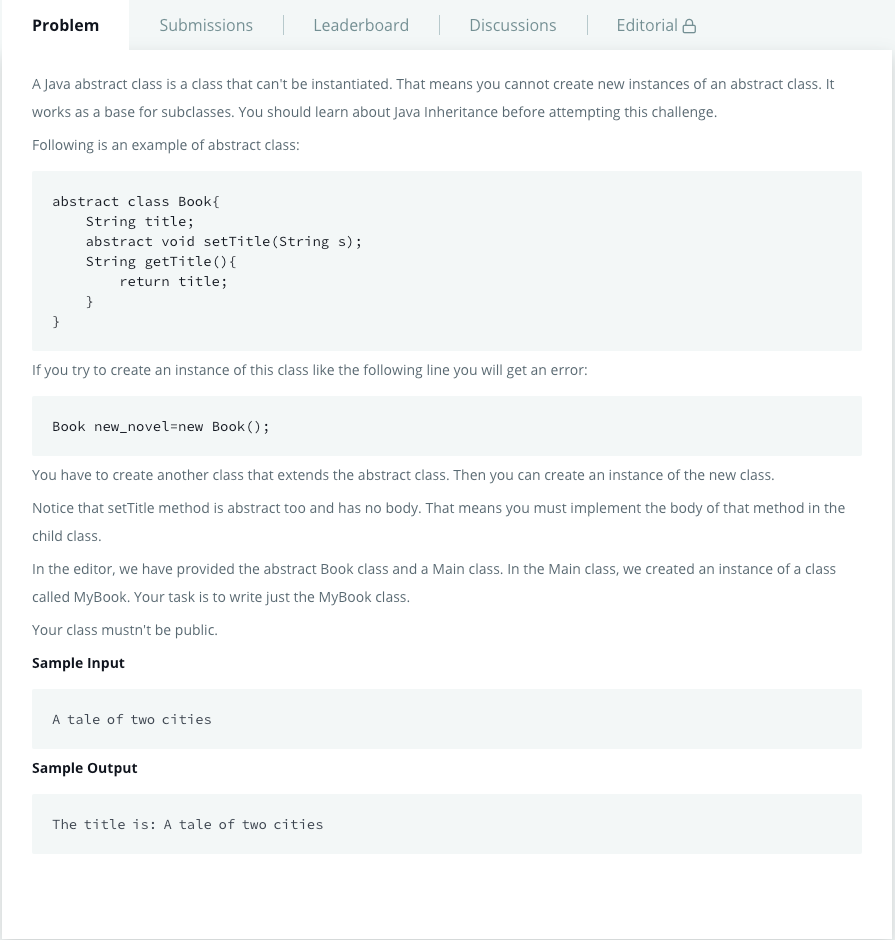Java Abstract Class, retrieved from https://www.hackerrank.com/challenges/java-abstract-class/problem
`import java.util.*;abstract class Book{    String title;    abstract void setTitle(String s);    String getTitle(){        return title;    }}//Write MyBook class hereclass MyBook extends Book {    void setTitle(String str) {        title = str;    }}public class Main{    public static void main(String []args){        //Book new_novel=new Book(); This line prHMain.java:25: error: Book is abstract; cannot be instantiated        Scanner sc=new Scanner(System.in);        String title=sc.nextLine();        MyBook new_novel=new MyBook();        new_novel.setTitle(title);        System.out.println("The title is: "+new_novel.getTitle());        sc.close();    }}`

## Java InterfaceJava Interface, retrieved from https://www.hackerrank.com/challenges/java-interface/problem
`import java.util.*;interface AdvancedArithmetic{    int divisor_sum(int n);}//Write your code hereclass MyCalculator implements AdvancedArithmetic {    public int divisor_sum(int n) {        int sum = n;        for (int i = 1; i < n; i++) {            if (n%i == 0) {                sum += i;            }        }        return  sum;    }}class Solution{    public static void main(String []args){        MyCalculator my_calculator = new MyCalculator();        System.out.print("I implemented: ");        ImplementedInterfaceNames(my_calculator);        Scanner sc = new Scanner(System.in);        int n = sc.nextInt();        System.out.print(my_calculator.divisor_sum(n) + "\n");        sc.close();    }    /*     *  ImplementedInterfaceNames method takes an object and prints the name of the interfaces it implemented     */    static void ImplementedInterfaceNames(Object o){        Class[] theInterfaces = o.getClass().getInterfaces();        for (int i = 0; i < theInterfaces.length; i++){            String interfaceName = theInterfaces[i].getName();            System.out.println(interfaceName);        }    }}`
`import java.util.*;interface AdvancedArithmetic{    int divisor_sum(int n);}//Write your code hereclass MyCalculator implements AdvancedArithmetic {    public int divisor_sum(int n) {        int sum = n;        for (int i = 1; i < n/2; i++) {            if (n%i == 0) {                sum += i;            }        }        return  sum;    }}class Solution{    public static void main(String []args){        MyCalculator my_calculator = new MyCalculator();        System.out.print("I implemented: ");        ImplementedInterfaceNames(my_calculator);        Scanner sc = new Scanner(System.in);        int n = sc.nextInt();        System.out.print(my_calculator.divisor_sum(n) + "\n");        sc.close();    }    /*     *  ImplementedInterfaceNames method takes an object and prints the name of the interfaces it implemented     */    static void ImplementedInterfaceNames(Object o){        Class[] theInterfaces = o.getClass().getInterfaces();        for (int i = 0; i < theInterfaces.length; i++){            String interfaceName = theInterfaces[i].getName();            System.out.println(interfaceName);        }    }}`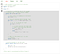Sum of all Divisors of a Natural Number, retrieved from https://www.geeksforgeeks.org/sum-of-all-proper-divisors-of-a-natural-number/

## Java Method Overriding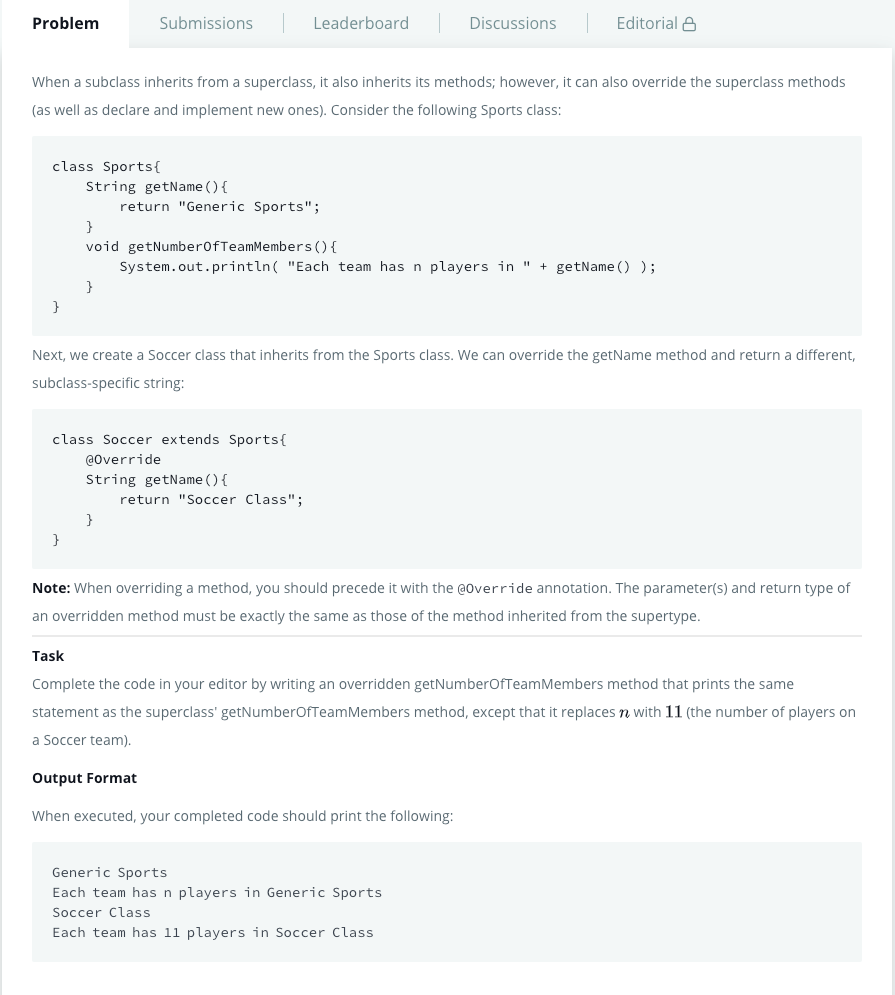Java Method Overriding, retrieved from https://www.hackerrank.com/challenges/java-method-overriding/problem
`import java.util.*;class Sports{    String getName(){        return "Generic Sports";    }    void getNumberOfTeamMembers(){        System.out.println( "Each team has n players in " + getName() );    }}class Soccer extends Sports{    @Override    String getName(){        return "Soccer Class";    }    // Write your overridden getNumberOfTeamMembers method here    void getNumberOfTeamMembers() {        System.out.println( "Each team has 11 players in " + getName() );    }}public class Solution{    public static void main(String []args){        Sports c1 = new Sports();        Soccer c2 = new Soccer();        System.out.println(c1.getName());        c1.getNumberOfTeamMembers();        System.out.println(c2.getName());        c2.getNumberOfTeamMembers();    }}`

## Java Method Overriding 2 (Super Keyword)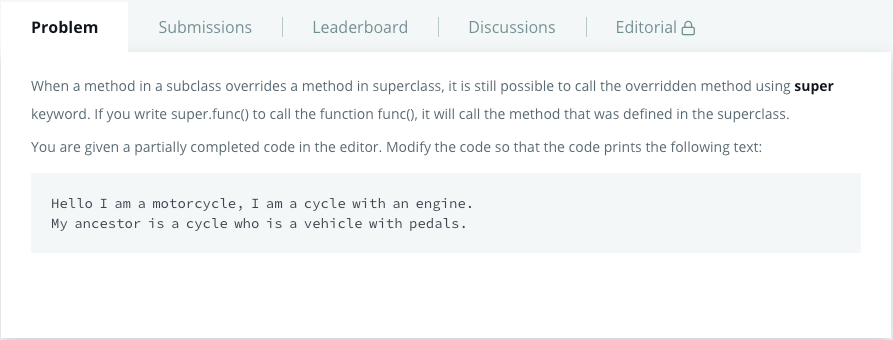Java Method Overriding 2 (Super Keyword), retrieved from https://www.hackerrank.com/challenges/java-method-overriding-2-super-keyword/problem
`import java.util.*;import java.io.*;class BiCycle{    String define_me(){        return "a vehicle with pedals.";    }}class MotorCycle extends BiCycle{    String define_me(){        return "a cycle with an engine.";    }    MotorCycle(){        System.out.println("Hello I am a motorcycle, I am "+ define_me());        String temp= super.define_me(); //Fix this line        System.out.println("My ancestor is a cycle who is "+ temp );    }}class Solution{    public static void main(String []args){        MotorCycle M=new MotorCycle();    }}`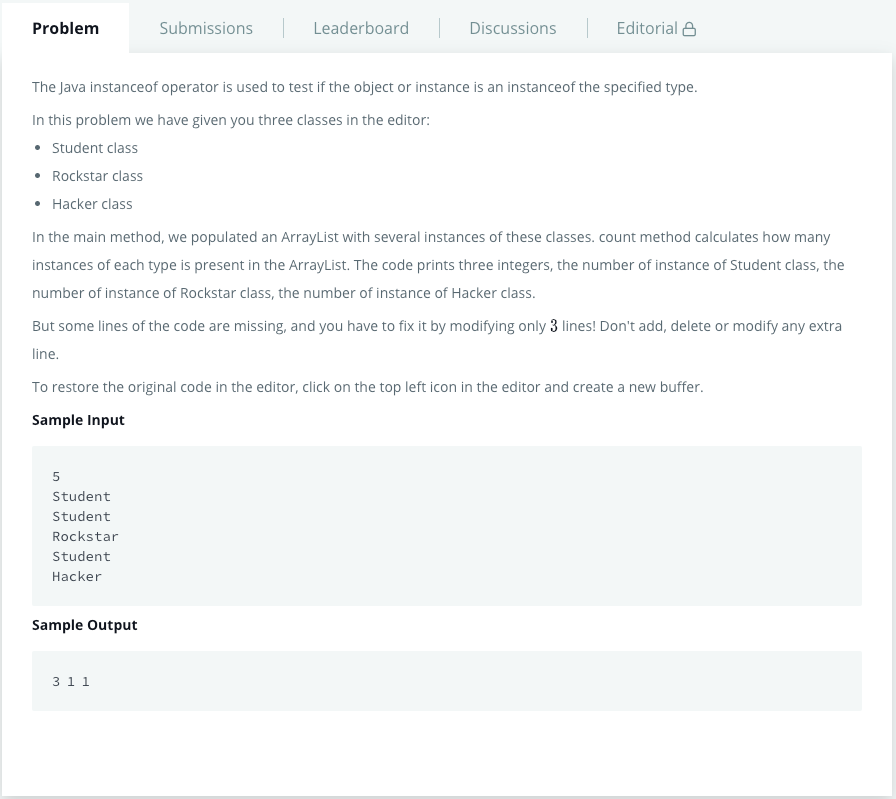Java Instanceof Keyword, retrieved from https://www.hackerrank.com/challenges/java-instanceof-keyword/problem
`import java.util.*;class Student{}class Rockstar{   }class Hacker{}public class InstanceOFTutorial{    static String count(ArrayList mylist){        int a = 0,b = 0,c = 0;        for(int i = 0; i < mylist.size(); i++){            Object element=mylist.get(i);            if(~~Complete this line~~)            a++;            if(~~Complete this line~~)            b++;            if(~~Complete this line~~)            c++;        }        String ret = Integer.toString(a)+" "+ Integer.toString(b)+" "+ Integer.toString(c);        return ret;    }    public static void main(String []args){        ArrayList mylist = new ArrayList();        Scanner sc = new Scanner(System.in);        int t = sc.nextInt();        for(int i=0; i<t; i++){            String s=sc.next();            if(s.equals("Student"))mylist.add(new Student());            if(s.equals("Rockstar"))mylist.add(new Rockstar());            if(s.equals("Hacker"))mylist.add(new Hacker());        }        System.out.println(count(mylist));    }}`
`import java.util.*;class Student{}class Rockstar{   }class Hacker{}public class InstanceOFTutorial{    static String count(ArrayList mylist){        int a = 0,b = 0,c = 0;        for(int i = 0; i < mylist.size(); i++){            Object element=mylist.get(i);            if(element instanceof Student)            a++;            if(element instanceof Rockstar)            b++;            if(element instanceof Hacker)            c++;        }        String ret = Integer.toString(a)+" "+ Integer.toString(b)+" "+ Integer.toString(c);        return ret;    }    public static void main(String []args){        ArrayList mylist = new ArrayList();        Scanner sc = new Scanner(System.in);        int t = sc.nextInt();        for(int i=0; i<t; i++){            String s=sc.next();            if(s.equals("Student"))mylist.add(new Student());            if(s.equals("Rockstar"))mylist.add(new Rockstar());            if(s.equals("Hacker"))mylist.add(new Hacker());        }        System.out.println(count(mylist));    }}`

## Java Iterator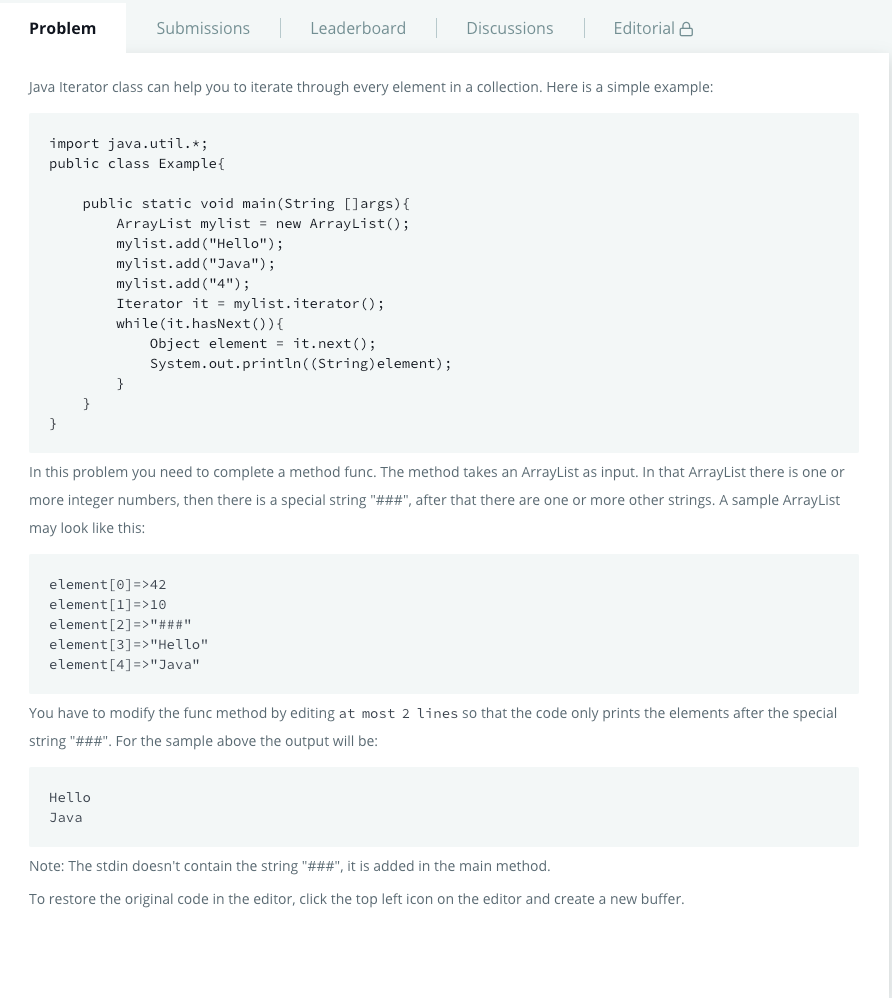Java Iterator, retrieved from https://www.hackerrank.com/challenges/java-iterator/problem
`import java.util.*;public class Main{    static Iterator func(ArrayList mylist){        Iterator it=mylist.iterator();        while(it.hasNext()){            Object element = it.next();            if(element instanceof String)//Hints: use instanceof operator                break;        }        return it;    }    @SuppressWarnings({ "unchecked" })    public static void main(String []args){        ArrayList mylist = new ArrayList();        Scanner sc = new Scanner(System.in);        int n = sc.nextInt();        int m = sc.nextInt();        for(int i = 0;i<n;i++){            mylist.add(sc.nextInt());        }        mylist.add("###");        for(int i=0;i<m;i++){            mylist.add(sc.next());        }        Iterator it=func(mylist);        while(it.hasNext()){            Object element = it.next();            System.out.println((String)element);        }    }}`

## Conclusion:

Undergraduate student / Research assistant/ Always curious / Opinions are mine

## More from Andrew Chen

Undergraduate student / Research assistant/ Always curious / Opinions are mine